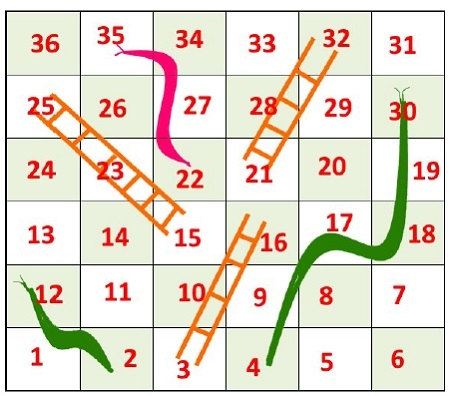We know about the famous game Snake and Ladder. In this game, some rooms are present on the board, with the room number. Some rooms are connected with a ladder or with snakes. When we get a ladder, we can climb up to some rooms to reach near to the destination without moving sequentially. Similarly, when we get some snake, it sends us to a lower room to start the journey again from that room.In this problem, we have to find the minimum number of the dice throw is required to reach start to destination.

## Input and Output

Input:
The starting and ending location of the snake and ladders.
Snake: From 26 to 0, From 20 to 8, From 16 to 3, From 18 to 6
Ladder From 2 to 21, From 4 to 7, From 10 to 25, from 19 to 28
Output:
Min Dice throws required is 3

## Algorithm

minDiceThrow(move, cell)

Input: jump location for snake or ladder, and the total number of cells.
Output: Minimum number of dice throw required to reach to the final cell.

Begin
initially mark all cell as unvisited
define queue q
mark the staring vertex as visited

for starting vertex the vertex number := 0 and distance := 0
add starting vertex s into q
while q is not empty, do
qVert := front element of the queue
v := vertex number of qVert
if v = cell -1, then //when it is last vertex
break the loop
delete one item from queue
for j := v + 1, to v + 6 and j < cell, increase j by 1, do
if j is not visited, then
newVert.dist := (qVert.dist + 1)
mark v as visited
if there is snake or ladder, then
else
newVert.vert := j
insert newVert into queue
done
done
return qVert.dist
End

## Example

#include<iostream>
#include <queue>
using namespace std;

struct vertex {
int vert;
int dist;       // Distance of this vertex from source
};

int minDiceThrow(int move[], int cell) {
bool visited[cell];
for (int i = 0; i < cell; i++)
visited[i] = false;    //initially all cells are unvisited

queue<vertex> q;

visited = true;       //initially starting from 0
vertex s = {0, 0};
q.push(s);             // Enqueue 0'th vertex

vertex qVert;
while (!q.empty()) {
qVert = q.front();
int v = qVert.vert;

if (v == cell-1)    //when v is the destination vertex
break;

q.pop();
for (int j=v+1; j<=(v+6) && j<cell; ++j) {    //for next 1 to 6 cells
if (!visited[j]) {
vertex newVert;
newVert.dist = (qVert.dist + 1);       //initially distance increased by 1
visited[j] = true;

if (move[j] != -1)
newVert.vert = move[j];       //if jth place have snake or ladder
else
newVert.vert = j;
q.push(newVert);
}
}
}
return qVert.dist;     //number of minimum dice throw
}

int main() {
int cell = 30;       //consider there are 30 cells
int moves[cell];

for (int i = 0; i<cell; i++)
moves[i] = -1;          //initially no snake or ladder are initialized

//For ladder in cell i, it jumps to move[i]
moves = 21;
moves = 7;
moves = 25;
moves = 28;

//For snake in cell i, it jumps to move[i]
moves = 0;
moves = 8;
moves = 3;
moves = 6;

cout << "Min Dice throws required is " << minDiceThrow(moves, cell);
}

## Output

Min Dice throws required is 3